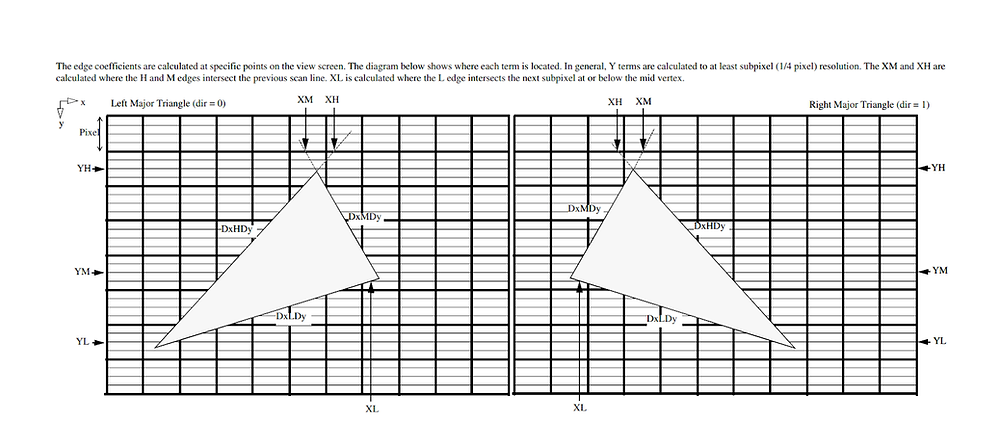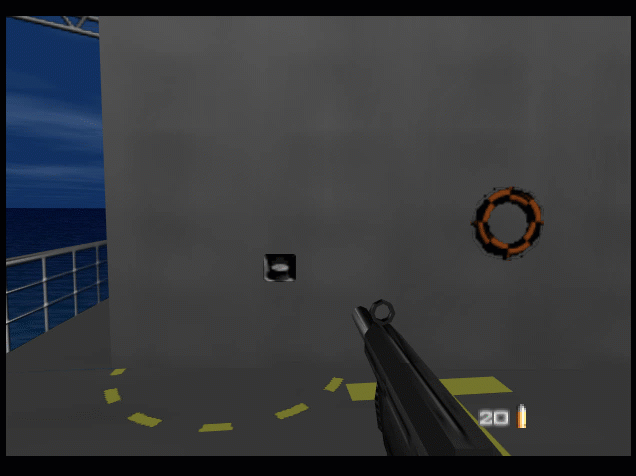# Rendering in Low Level Emulation mode. Part II

Hi!

In the previous article I described how N64 hardware does polygons rasterization and how graphics plugins convert low-level rasterization data into vertices for PC hardware. I also mentioned the problems of the current approach. So, the task is to solve the problems.

When I finally understood how current code for low-level triangles works (yes, I had borrowed it years ago without understanding of its work), I had no good idea why its results are so poor. Everything looked quite reasonable.

When I have problems with understanding how the RDP works, I consult with sources of angrylion's software graphics plugin aka AL RDP. This plugin is very precise emulator of RDP, a digital model of the actual chip. The sources can give you information about RDP work, which you will not find in documentations. Extraction of information this way is not easy but often it is the shortest way. I found how edge walking implemented here and compared it with GLideN64 code. No surprises here - AL RDP code is more complex and precise, it does many more checks and manipulations with input data and output result. So I decided to adapt that code for my needs in hope that it will bring better results. I spend a week or two on experiments but got as bad or even worse results as before. Most likely I did mistakes somewhere. Anyway, my disappointments in results turned into disappointments in the approach itself.

At some moment I got an idea: why should we walk the same way as actual hardware does? We have three edges, we need to find where they are intersecting. This is simpler to do with just solving lines equations! Actually, this idea came to olivieryuyu first. He started to learn that matter earlier and provided me with articles and documents how things work and how low-level data can be used to extract vertices. I did not pay attention on it first, trying to improve the old method, which already works somehow.

So, let's see how can we get vertices without edge walking. We need the line equation for each edge. Equation of a line looks as this:

y = mx + y0

where m is the slope and y0 is the y coordinate of its intersection with the y-axis.

Open our diagram for low-level triangles again:We have inverse slope for each edge, so the equation of a line in our case is

x = ky + x0

where k is edge's inverse slope and x0 is the x coordinate of its intersection with the x-axis.

We have intersection point for the major edge H, which is XH. We also have intersection point for the first minor edge M, which is XM. Both intersection points have the same y coordinate, which is coordinate of the previous scan-line. Let's set y-axis to that y coordinate for convenience and denote it as Y0.

Thus, we have two equations:

x = DxHDy * y + XH

x = DxMDy * y + MH

where y = (Y - Y0).

We can easily solve the system of these two equations and get exact coordinates of intersection point of the edges. Good. Let's denote coordinates of that point as (X1,Y1).

How to get parameters values for that point? It is simple: with DpDe.

Value of P at (X1,Y1) is

P1 = P + DpDe * (Y1 - Y0)

Next vertex is intersection point of minor edges M and L. As you see on the diagram, y coordinate of mid vertex is in range [YM,YM+1]. XL also does not coincide with vertex x coordinate in general case. L edge intersects XL, but where? "XL is calculated where the L edge intersects the next subpixel at or below the mid vertex." The intersection can be at YL or YL+1. Can we calculate it exactly? It looks as we can. Let's calculate XM` for edge M at YM:

XM' = DxMDy * YM + MH

If XM' is the same as XL then mid vertex coordinates are (XL,YM).

Otherwise, edge L intersects point (XL,YM+1), so we can build line equation for L using this point, and then find intersection point of M and L edges. Let's denote mid vertex coordinates as (X2,Y2).

Now we know coordinates of the second vertex, but the vertex is not on the major edge. How will we get values of all parameters for that vertex? It is not hard either:

• find the point on the major edge at mid vertex Y, that is Y2. Let's name x coordinate of that point as XH', so the point's coordinates are (XH',Y2).

• calculate parameters for point (XH',Y2) using DpDe.

• We know X2 and XH', so we can calculate the distance X2-XH', and thus we can calculate value of every parameter with DpDx: P2 = P + DpDe * (Y2 - Y0) + DpDx * (X2 - XH')

Since we have line equations for all three edges, we can find intersection point of the major edge H and minor edge L, which is the third vertex of our triangle. This vertex lies on edge H, so its parameters calculated the same way as for the first vertex. Thus, we've got three vertices per low-level triangle. The task completed.

The algorithm looks simple, does not it? I made first-shot implementation pretty quickly, but then I spent weeks trying to make it work at least not worse than the older method, and some more time to make it better. The devil in the details.

Which problems of the old method are solved with the new one

• Performance. New method produces 3 vertices and thus 1 hardware triangle per low-level triangle. When low-level triangle represents trapezoid, it still requires 4 vertices and thus two hardware triangles, but it is a rare case. Thus, the new method produces significantly less data for PC hardware than the old one. I would be happy to say that it led to significant boost in performance, but it is not true. The difference in performance is almost negligible. The bottle-neck of LLE rendering is not in amount of data and number of polygons, it is in number of API calls. Each LLE triangle rendered with separate GL draw call, while in HLE mode the plugin caches triangles when possible. I had no idea how to implement triangles caching for LLE mode, and, truly speaking, did not care much about it. This time I looked at the problem more carefully and found a simple but efficient way to cache LLE triangles. The speed-up after that optimization can be called tremendous. Now GLideN64 is even faster than Z64, which is also well optimized.

• Lines support. As I explained before, line is a special case of low-level triangle, and it requires a special support in the code. It was not done for the old method, so lines rendered incorrectly. I've implemented support for special cases for the new method:It is still not perfect if compare with HLE mode lines, and I hope to improve it.

• Sticking out polygons. I see them no more:• Depth compare issues. Some poke-through issues have been fixed:• Depth buffer emulation issues. I adapted my software depth render to work with new LLE triangles. Depth buffer based effects such as coronas now work correct, as you may see on the previous screen shot.

Unsolved problems

• Poke-through issue with decal surfaces. Decal textures worked poorly with old method and still work the same with the new one:I still don't know where the problem hides.

• Gaps between polygons. No positive changes here. I just hope that the new method brings no regressions in compare with the old one.

And the most interesting from the practical view question: does the new method help to fix issues with Golden Eye and Killer Instinct Gold? No, it does not.

To be continued.

Original published at Blogspot

Main Page